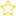Resources for teaching statistics: Key Resources, Teacher Preparation

For any type of situation, we investigate whether bootstrap confidence intervals work (or whether any other type of confidence intervals work) using “computer simulation”. Seeing this in action also gives us a better understanding of what confidence intervals are.

After you’ve watched this video, you should be able to answer these questions:

• What is the basic idea of how a bootstrap confidence interval is constructed to capture the true population value of some quantity (e.g. a mean, a median, a percentage, ..)?
• What do we do to find out whether a method for constructing confidence intervals works?
• What do we mean by “our interval has covered the true value”?
• What do we know about bootstrapping as a way of constructing confidence intervals?
• What is meant when we say that a particular interval is a “95% confidence interval”?

If you couldn’t answer a question, you might find it helpful to look at the Illustrated transcript.

## Rate this resource:(No ratings yet)